## A 7.35 H inductor with negligible resistance is placed in series with a 14.1 V battery, a 3.00 ? resistor, and a switch. The switch is close

Question

A 7.35 H inductor with negligible resistance is placed in series with a 14.1 V battery, a 3.00 ? resistor, and a switch. The switch is closed at time t = 0 seconds.

(a) Calculate the initial current at t = 0 seconds.

(b) Calculate the current as time approaches infinity.

(c) Calculate the current at a time of 6.25 s.

(d) Determine how long it takes for the current to reach half of its maximum.

in progress 0
3 weeks 2021-09-03T17:09:44+00:00 1 Answers 0 views 0

(a). The initial current at t=0 is zero.

(b). The current as time approaches infinity is 4.7 A

(c). The current at a time of 6.25 s is 4.33 A.

(d). The time is 1.69 sec.

Explanation:

Given that,

Inductance = 7.35 H

Voltage = 14.1 V

Resistance= 3.00 ohm

(a). We need to calculate the initial current at t = 0

Using formula of current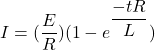Put the value into the formula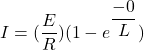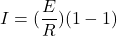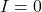(b). We need to calculate the current as time approaches infinity.

Using formula of currentPut the value into the formula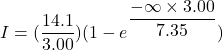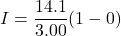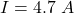(c).  We need to calculate the current at a time of 6.25 s

Using formula of currentPut the value into the formula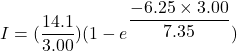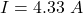(d). We need to calculate the time

Using  formula of currentPut the value into the formula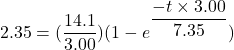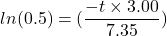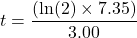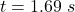Hence, (a). The initial current at t=0 is zero.

(b). The current as time approaches infinity is 4.7 A

(c). The current at a time of 6.25 s is 4.33 A.

(d). The time is 1.69 sec.